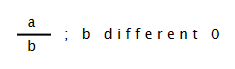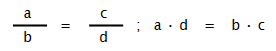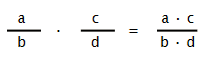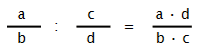# Fractions

#### Video Lesson on Fractions

Fraction: It represents a part of a whole or more generally, any number of equal parts. If a unit is divided into any number of equal parts, then one or more of these parts is termed as a fraction of the unit.
A fraction is the quotient of two integers a and b, which is represented as follows:bioprofe |exams with exercises about physics, chemistry and mathematics | Fractions
b -> denominator, indicates the number of parties that has been split the unit.
a -> numerator, indicates the number of fractional units chosen.

## Types of fractions

Fractions can be proper or improper. Proper fractions are those whose numerator is less than the denominator, therefore, its value is between zero and one. Moreover, improper fractions are those whose numerator is greater than the denominator, that is, its value is greater than 1.
In turn we have the following types of fractions:
Decimal fractions:Such fractions have as denominator a power of 10.
Equivalent fractions: Two fractions are equivalent when the end product is the same as product of media.Note:a and d are the ends and b and c are the means.## Simplifying fractions

Simplifying a fraction is to transform a fraction into another which is equivalent and simpler.

• To simplify a fraction we divide numerator and denominator by the same number.
• We begin to simplify testing for prime numbers: 2, 3, 5 … I mean, we try to divide the numerator and denominator between 2 while you can, then move on to 3 and so on.
• The process is repeated until there is no more common divisors.
• If the terms of the fraction ending in zeros, we start by removing the common zeros end from the numerator and denominator, which is equivalent to dividing numerator and denominator by the same power of 10.
• If the number you divide is the greatest common divisor of the numerator and denominator we have the irreducible fraction.

### Irreducible fractions

The irreducible fractions are those that can not be simplified, this happens when the numerator and denominator are relatively prime, or what is the same, when the GCD of two numbers is 1.

### Order fractions

To order two or more fractions and, therefore, to know which is greater or less than another, we must first note that they have the same denominator. If they have the same denominator we just compare the numerators, if it were not so, we must reduce them to a common denominator by calculating the mcm of the denominators and then compare the numerators. If two or more fractions have different denominators but the same numerator, it will be greater the one that has a lower denominator.

## Operations with fractions

### Add and subtract:

To make both a sum as a subtraction we must look at that all the fractions have the same denominator, if so, add or subtract the numerators and the result will be the new number in the denominator that is common in all fractions. If not, it is said not having the same denominator, we must make common denominator by calculating the mcm of the denominators and then perform the operation.

### Multiplying fractions:

From the multiplication between two or more fractions we obtain another fraction with the numerator is the result of the product of the numerators and denominators is the result of the product of the denominators, it is said:### Dividing fractions:

From the division between two fractions we obtain another fraction with the numerator the result of the end product and denominator the result of media product, it is said:## Generatrix Fraction

An exact decimal number or periodic number can be expressed as a fraction, called fraction generatrix of the following ways:

### Pass from exact decimal to a fraction:

If the exact decimal fraction is the fraction that has as numerator the number given without the comma, and denominator, the unit followed by as many zeros as decimal places have.

### Pass from pure periodic to generatrix fraction:

If the fraction is periodic pure, the generating fraction has as numerator the number given without the comma, minus the integer part, and denominator consists in many nines number as numbers has the period.

### Pass from mixed periodic to generatrix fraction:

If the fraction is mixed periodically, the generating fraction has as numerator the number given without the comma, minus the integer part followed by non-recurrent decimal places, and for denominator a number formed by many nines as numbers has the period, followed by so much zeros as the non-periodic part decimal has.

### About us

EntryTest.com is a free service for students seeking successful career.CAT - College of Admission Tests. All rights reserved. College of Admission Tests Online Test Preparation The CAT Online